# A students work without solutions manual 10 problemsnight

• Slides: 67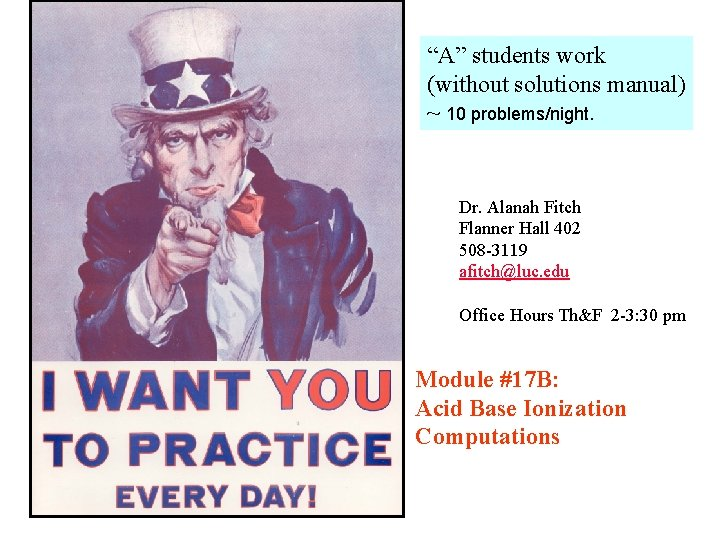“A” students work (without solutions manual) ~ 10 problems/night. Dr. Alanah Fitch Flanner Hall 402 508 -3119 [email protected] edu Office Hours Th&F 2 -3: 30 pm Module #17 B: Acid Base Ionization Computations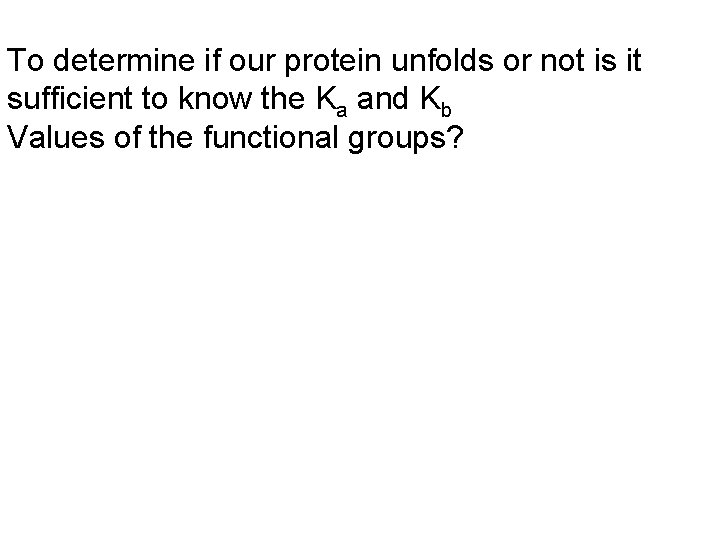To determine if our protein unfolds or not is it sufficient to know the Ka and Kb Values of the functional groups?2 1 3 D structure of ALAD directs Reactants into proper orientation 3 D structure controlled by proper hydrogen and ionic bonds, p. H dependent!!!!!!!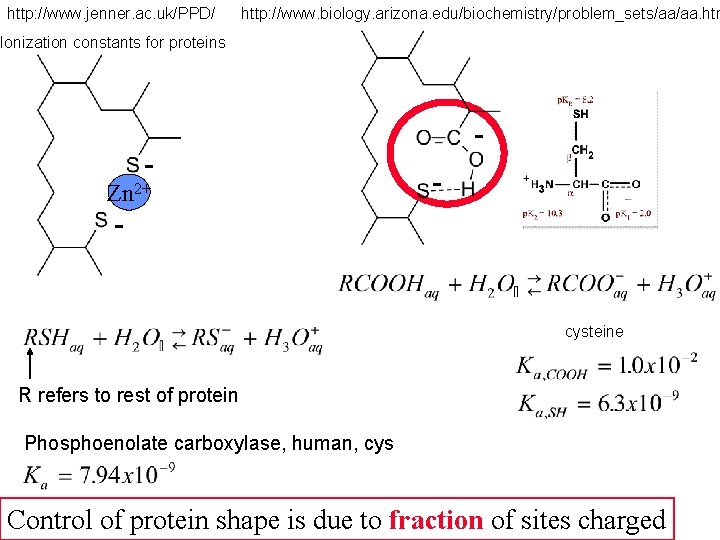http: //www. jenner. ac. uk/PPD/ http: //www. biology. arizona. edu/biochemistry/problem_sets/aa/aa. htm Ionization constants for proteins - Zn 2+ - - cysteine R refers to rest of protein Phosphoenolate carboxylase, human, cys Control of protein shape is due to fraction of sites charged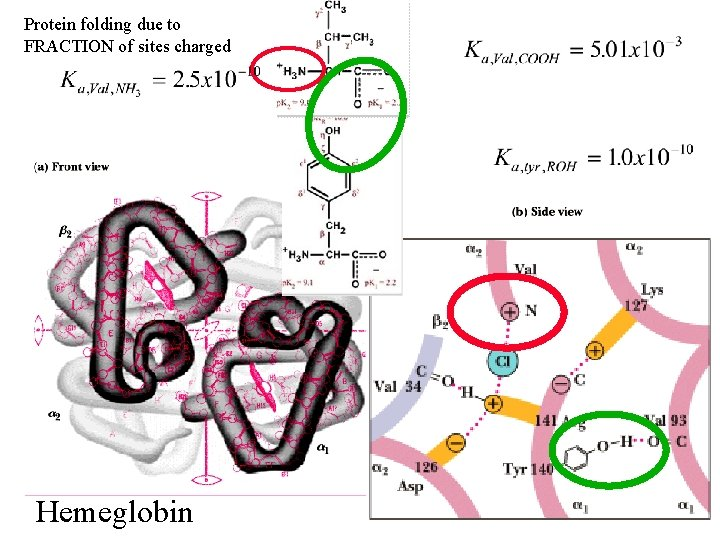Protein folding due to FRACTION of sites charged Hemeglobin% Ionized (dissociated) This seems pretty straight forward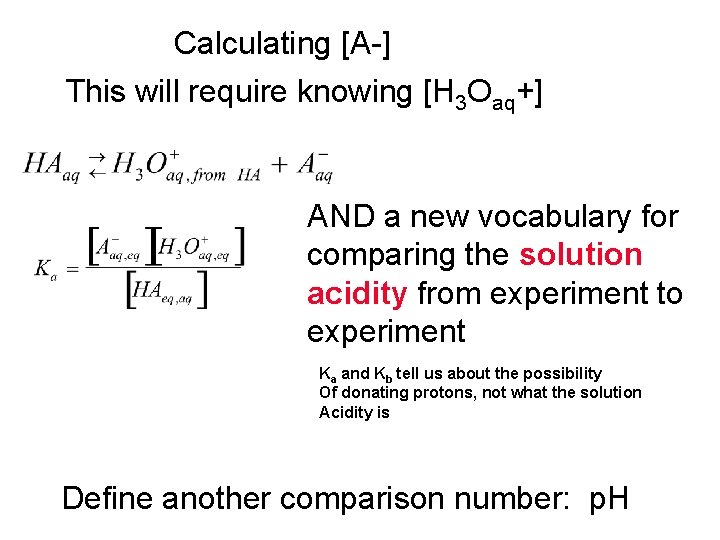Calculating [A-] This will require knowing [H 3 Oaq+] AND a new vocabulary for comparing the solution acidity from experiment to experiment Ka and Kb tell us about the possibility Of donating protons, not what the solution Acidity is Define another comparison number: p. H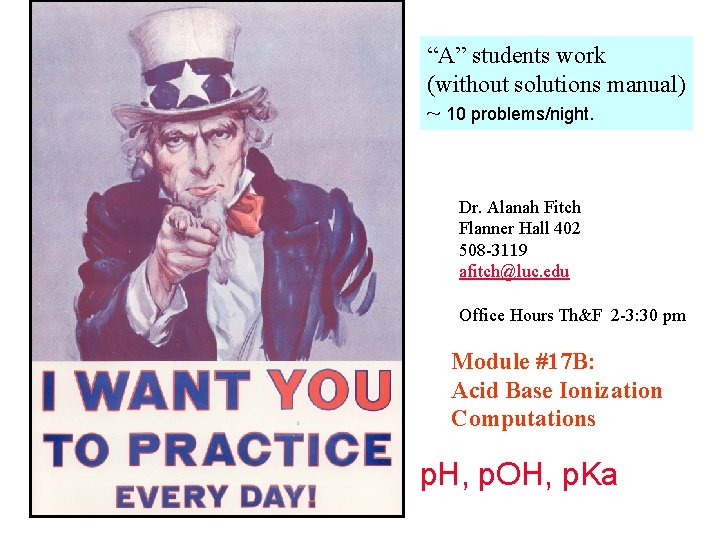“A” students work (without solutions manual) ~ 10 problems/night. Dr. Alanah Fitch Flanner Hall 402 508 -3119 [email protected] edu Office Hours Th&F 2 -3: 30 pm Module #17 B: Acid Base Ionization Computations p. H, p. OH, p. KaLAZY Chemists are _____ To shorten calculations use logAssumption that 55. 5 molar is relative unchanged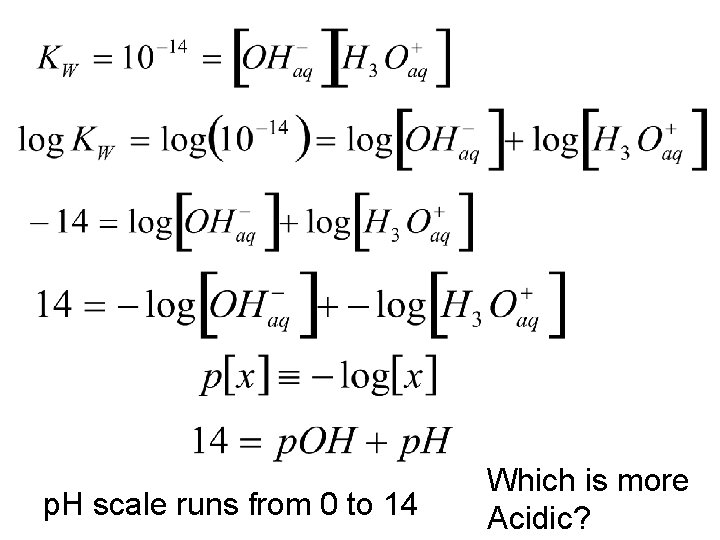p. H scale runs from 0 to 14 Which is more Acidic?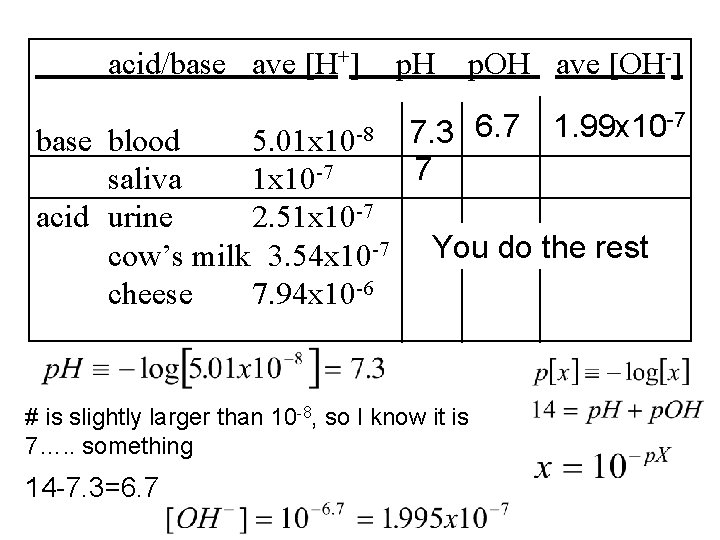acid/base ave [H+] base blood 5. 01 x 10 -8 saliva 1 x 10 -7 acid urine 2. 51 x 10 -7 cow’s milk 3. 54 x 10 -7 cheese 7. 94 x 10 -6 p. H p. OH ave [OH-] 7. 3 6. 7 7 You do the rest # is slightly larger than 10 -8, so I know it is 7…. . something 14 -7. 3=6. 7 1. 99 x 10 -7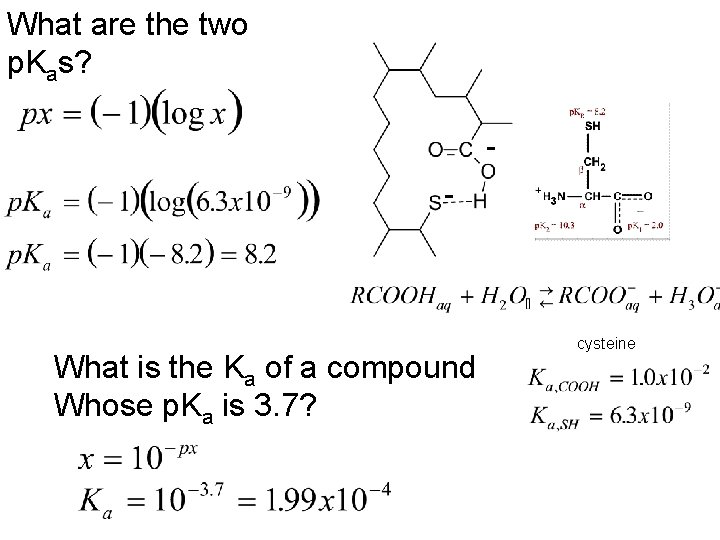What are the two p. Kas? - What is the Ka of a compound Whose p. Ka is 3. 7? cysteine“A” students work (without solutions manual) ~ 10 problems/night. Dr. Alanah Fitch Flanner Hall 402 508 -3119 [email protected] edu Office Hours Th&F 2 -3: 30 pm Module #17 B: Acid Base Ionization ComputationsCalculating [A-] This will require an equilibrium calculation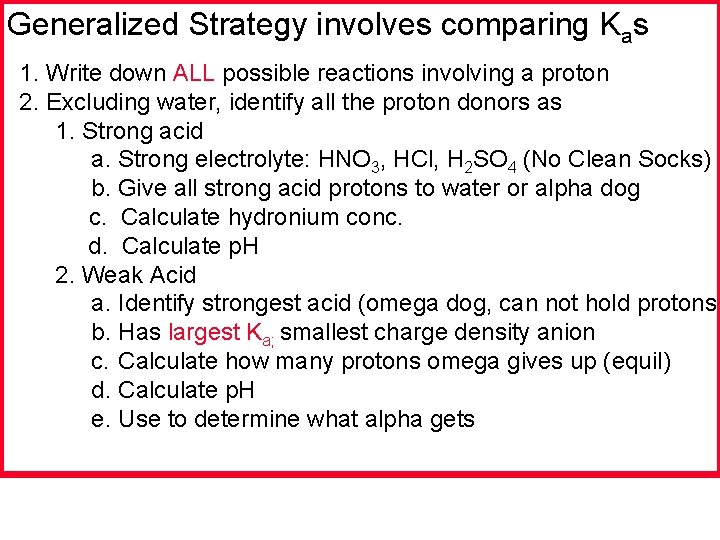Generalized Strategy involves comparing Kas 1. Write down ALL possible reactions involving a proton 2. Excluding water, identify all the proton donors as 1. Strong acid a. Strong electrolyte: HNO 3, HCl, H 2 SO 4 (No Clean Socks) b. Give all strong acid protons to water or alpha dog c. Calculate hydronium conc. d. Calculate p. H 2. Weak Acid a. Identify strongest acid (omega dog, can not hold protons) b. Has largest Ka; smallest charge density anion c. Calculate how many protons omega gives up (equil) d. Calculate p. H e. Use to determine what alpha gets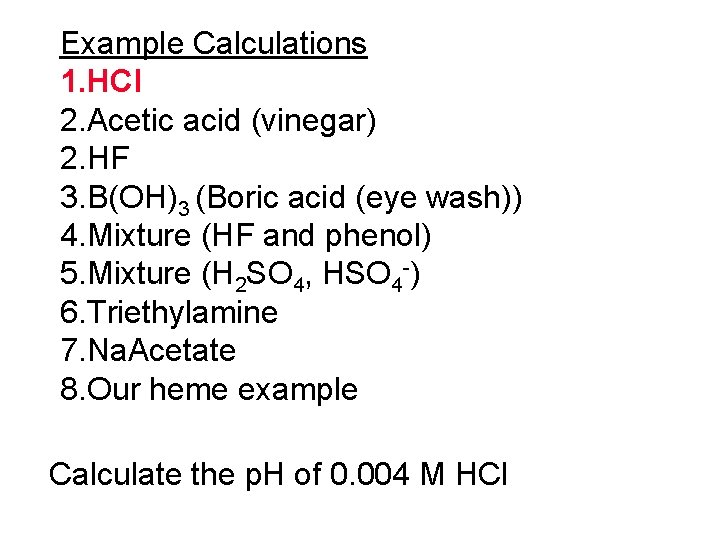Example Calculations 1. HCl 2. Acetic acid (vinegar) 2. HF 3. B(OH)3 (Boric acid (eye wash)) 4. Mixture (HF and phenol) 5. Mixture (H 2 SO 4, HSO 4 -) 6. Triethylamine 7. Na. Acetate 8. Our heme example Calculate the p. H of 0. 004 M HClGeneralized Strategy involves comparing Kas 1. Write down ALL possible reactions involving a proton 2. Excluding water, identify all the proton donors as 1. Strong acid a. Strong electrolyte: HNO 3, HCl, H 2 SO 4 (No Clean Socks) Omega dog b. Give all strong acid protons to water or alpha present c. Calculate hydronium conc. d. Calculate p. H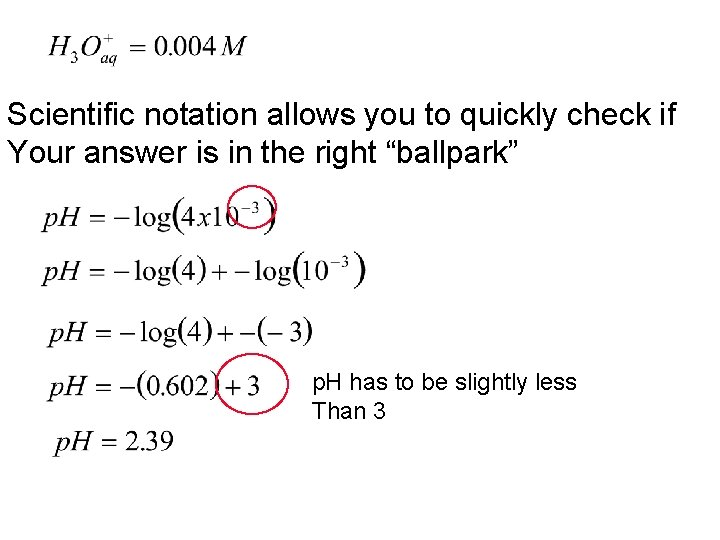Scientific notation allows you to quickly check if Your answer is in the right “ballpark” p. H has to be slightly less Than 3Example Calculations 1. HCl 2. Acetic acid (vinegar) 2. HF 3. B(OH)3 (Boric acid (eye wash)) 4. Mixture (HF and phenol) 5. Mixture (H 2 SO 4, HSO 4 -) 6. Triethylamine 7. Na. Acetate 8. Our heme example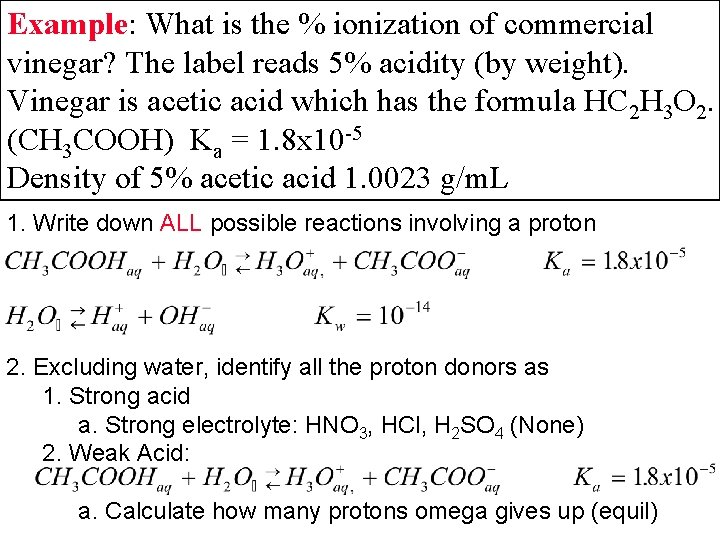Example: What is the % ionization of commercial vinegar? The label reads 5% acidity (by weight). Vinegar is acetic acid which has the formula HC 2 H 3 O 2. (CH 3 COOH) Ka = 1. 8 x 10 -5 Density of 5% acetic acid 1. 0023 g/m. L 1. Write down ALL possible reactions involving a proton 2. Excluding water, identify all the proton donors as 1. Strong acid a. Strong electrolyte: HNO 3, HCl, H 2 SO 4 (None) 2. Weak Acid: a. Calculate how many protons omega gives up (equil)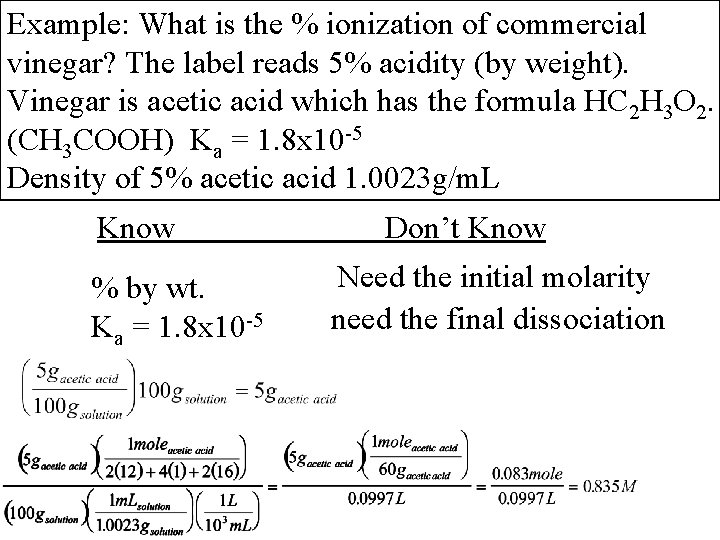Example: What is the % ionization of commercial vinegar? The label reads 5% acidity (by weight). Vinegar is acetic acid which has the formula HC 2 H 3 O 2. (CH 3 COOH) Ka = 1. 8 x 10 -5 Density of 5% acetic acid 1. 0023 g/m. L Know % by wt. Ka = 1. 8 x 10 -5 Don’t Know Need the initial molarity need the final dissociation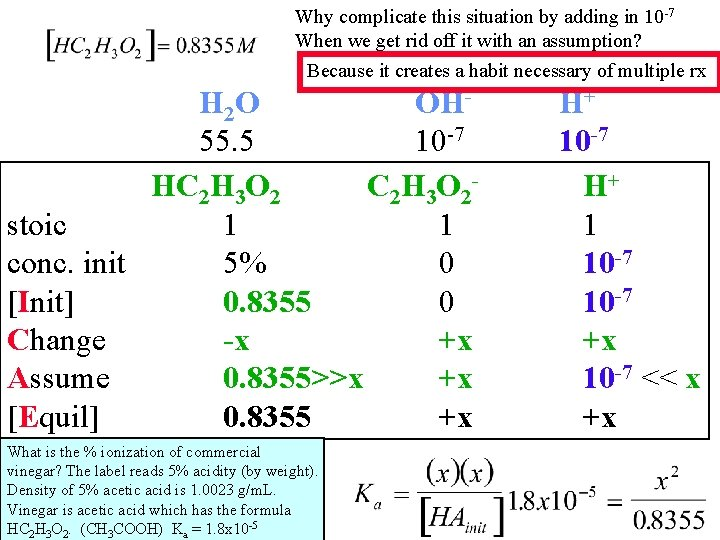Why complicate this situation by adding in 10 -7 When we get rid off it with an assumption? Because it creates a habit necessary of multiple rx H 2 O OH 55. 5 10 -7 HC 2 H 3 O 2 stoic 1 1 conc. init 5% 0 [Init] 0. 8355 0 Change -x +x Assume 0. 8355>>x +x [Equil] 0. 8355 +x What is the % ionization of commercial vinegar? The label reads 5% acidity (by weight). Density of 5% acetic acid is 1. 0023 g/m. L. Vinegar is acetic acid which has the formula HC 2 H 3 O 2. (CH 3 COOH) Ka = 1. 8 x 10 -5 H+ 10 -7 H+ 1 10 -7 +x 10 -7 << x +xHA 0. 8355>>x Assume A +x H+ 10 -7 << x Original sig figs were = 0. 83 So if we round to 2 sig fig, have Same answer Check: + p. H =-log(0. 003878)= 2. 41 Sig figs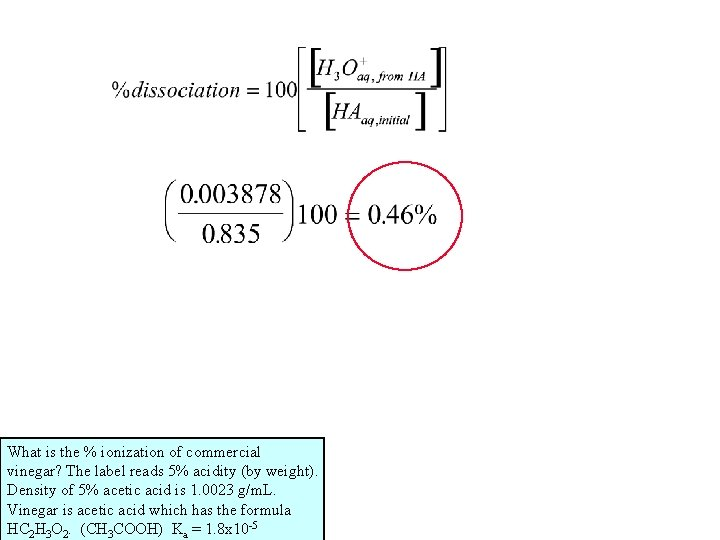What is the % ionization of commercial vinegar? The label reads 5% acidity (by weight). Density of 5% acetic acid is 1. 0023 g/m. L. Vinegar is acetic acid which has the formula HC 2 H 3 O 2. (CH 3 COOH) Ka = 1. 8 x 10 -5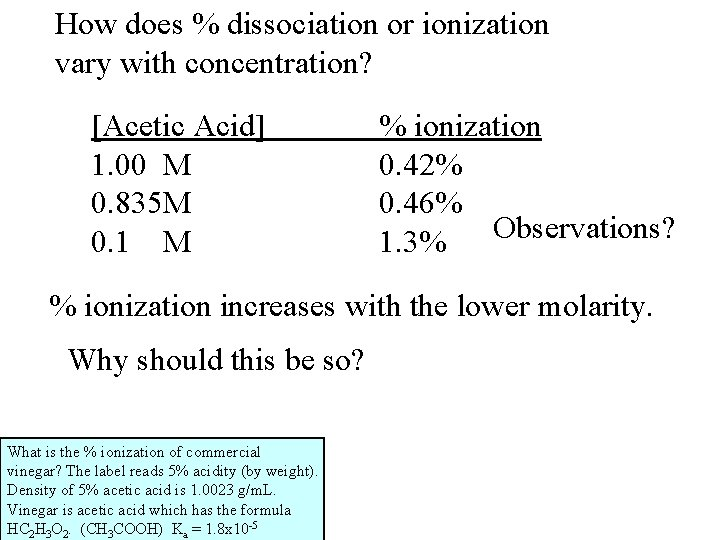How does % dissociation or ionization vary with concentration? [Acetic Acid] 1. 00 M 0. 835 M 0. 1 M % ionization 0. 42% 0. 46% 1. 3% Observations? % ionization increases with the lower molarity. Why should this be so? What is the % ionization of commercial vinegar? The label reads 5% acidity (by weight). Density of 5% acetic acid is 1. 0023 g/m. L. Vinegar is acetic acid which has the formula HC 2 H 3 O 2. (CH 3 COOH) Ka = 1. 8 x 10 -5Dilute by 10 (make less concentrated): What does this tell us, if anything? We have too many reactants, need to shift to the right, or dissociate some more. general rule of thumb: dilution gives more dissociation.Example Calculations 1. HCl 2. Acetic acid (vinegar) 3. HF 4. B(OH)3 (Boric acid (eye wash)) (students Do this one yourself) 5. Mixture (HF and phenol) 6. Mixture (H 2 SO 4, HSO 4 -) 7. Triethylamine 8. Na. Acetate 9. Our heme exampleCalculate the F- of a solution of 1. 00 M HF. Ka= 7. 2 x 10 -4 1. Write down ALL possible reactions involving a proton 2. Excluding water, identify all the proton donors as 1. Strong acid a. Strong electrolyte: HNO 3, HCl, H 2 SO 4 (No Clean Socks) No Strong Acids (SA) 2. Weak Acid a. Identify strongest acid (omega dog, can not hold protons) b. Has largest Ka; smallest charge density anion c. Calculate how many protons omega gives up (equil)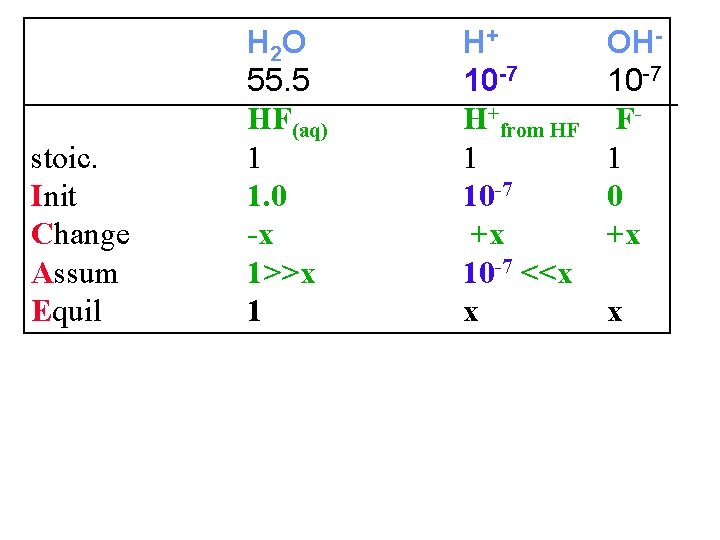stoic. Init Change Assum Equil H 2 O 55. 5 HF(aq) 1 1. 0 -x 1>>x 1 H+ 10 -7 H+from HF 1 10 -7 +x 10 -7 <<x x OH 10 -7 F 1 0 +x xInit Change Assum Equil HF(aq) 1. 0 -x 1>>x 1 H+from HF F 10 -7 0 +x +x 10 -7 <<x x x Check assumptions Calculate the p. H of a solution of 1. 00 M HF. K 1 = 7. 2 x 10 -4 Sig fig is here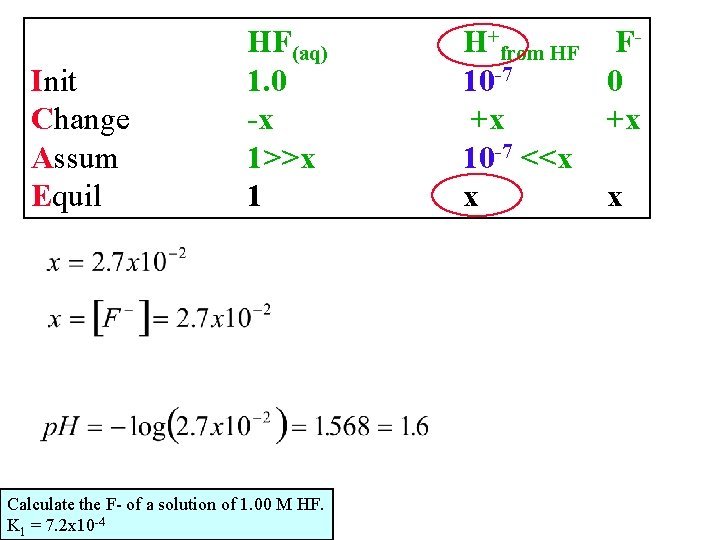Init Change Assum Equil HF(aq) 1. 0 -x 1>>x 1 Calculate the F- of a solution of 1. 00 M HF. K 1 = 7. 2 x 10 -4 H+from HF F 10 -7 0 +x +x 10 -7 <<x x xExample: Boric acid is commonly used in eyewash solutions to neutralize bases splashed in the eye. It acts as a monoprotic acid, but the dissociation reaction looks different. Calculate the p. H of a 0. 75 M solution of boric acid, and the concentration of B(OH)4 -. 1. Write down ALL possible reactions involving a proton 2. Identify proton donors 1. strong acids: No Clean Socks? 2. Weak acids: No SA Students do B(OH)3 This on your own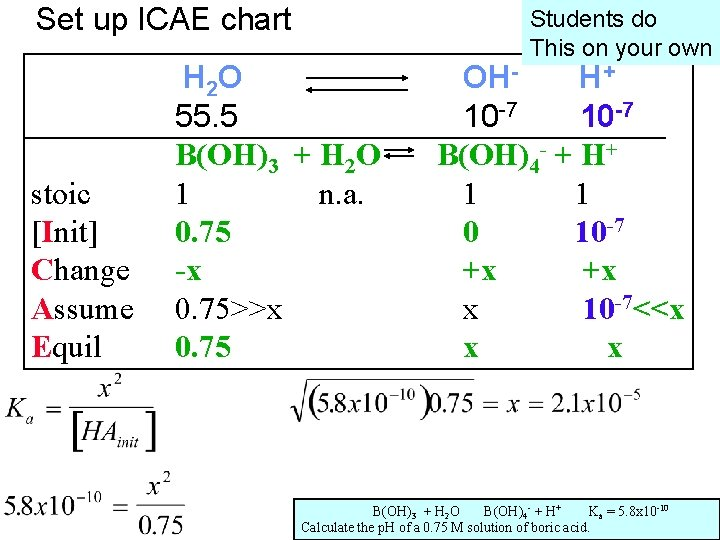Set up ICAE chart stoic [Init] Change Assume Equil Students do This on your own H 2 O 55. 5 B(OH)3 + H 2 O 1 n. a. 0. 75 -x 0. 75>>x 0. 75 check? ? two assumptions. B(OH) OHH+ 10 -7 B(OH)4 - + H+ 1 1 0 10 -7 +x +x x 10 -7<<x x x + H 2 O B(OH)4 - + H+ Ka = 5. 8 x 10 -10 Calculate the p. H of a 0. 75 M solution of boric acid. 3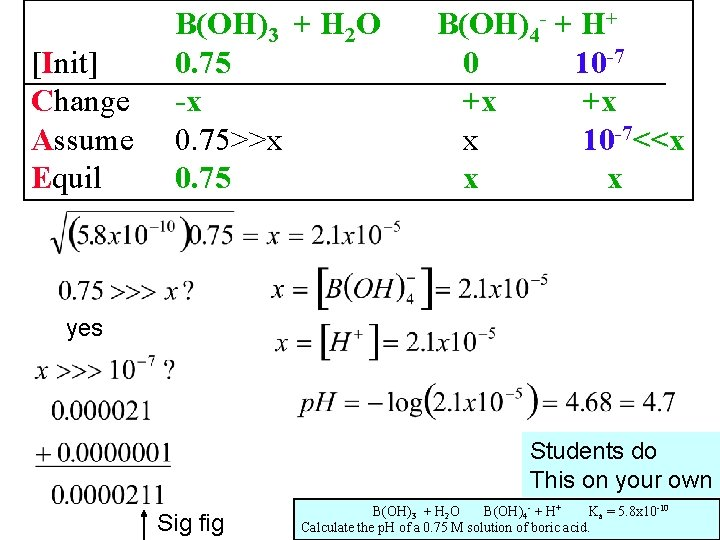[Init] Change Assume Equil B(OH)3 + H 2 O 0. 75 -x 0. 75>>x 0. 75 B(OH)4 - + H+ 0 10 -7 +x +x x 10 -7<<x x x yes check? ? two assumptions. B(OH) Sig fig Students do This on your own + H 2 O B(OH)4 - + H+ Ka = 5. 8 x 10 -10 Calculate the p. H of a 0. 75 M solution of boric acid. 3Example Calculations 1. HCl 2. Acetic acid (vinegar) 3. HF 4. B(OH)3 (Boric acid (eye wash)) (students Do this one yourself) 5. Mixture (HF and phenol) 6. Mixture (H 2 SO 4, HSO 4 -) 7. Triethylamine 8. Na. Acetate 9. Our heme exampleMixtures of Acids Calculate the p. H of a solution that contains 1. 0 M HF and 1. 0 M HOC 6 H 5. Calculate the conc. of -OC 6 H 5 at this concentration. 1. Write down ALL possible reactions involving a proton 2. Excluding water, identify all the proton donors as 1. Strong acid NONE 2. Weak Acid Identify strongest acid (omega dog, can not hold protons) Has largest Ka; smallest charge density anion Calculate how many protons omega gives up (equil) Calculate p. H (Use to determine what alpha gets)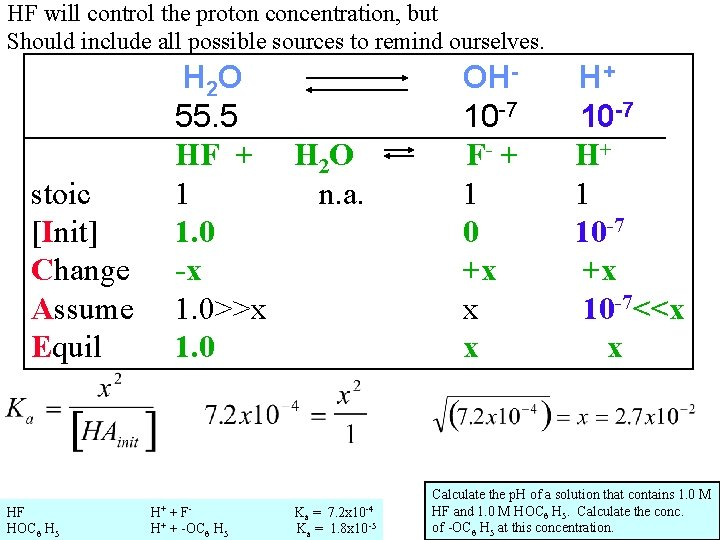HF will control the proton concentration, but Should include all possible sources to remind ourselves. H 2 O 55. 5 HF + H 2 O 1 n. a. 1. 0 -x 1. 0>>x 1. 0 stoic [Init] Change Assume Equil OH 10 -7 F- + 1 0 +x x x check? ? H two assumptions. +F K = 7. 2 x 10 HF HOC 6 H 5 + - H+ + -OC 6 H 5 a -4 Ka = 1. 8 x 10 -5 H+ 10 -7 H+ 1 10 -7 +x 10 -7<<x x Calculate the p. H of a solution that contains 1. 0 M HF and 1. 0 M HOC 6 H 5. Calculate the conc. of -OC 6 H 5 at this concentration.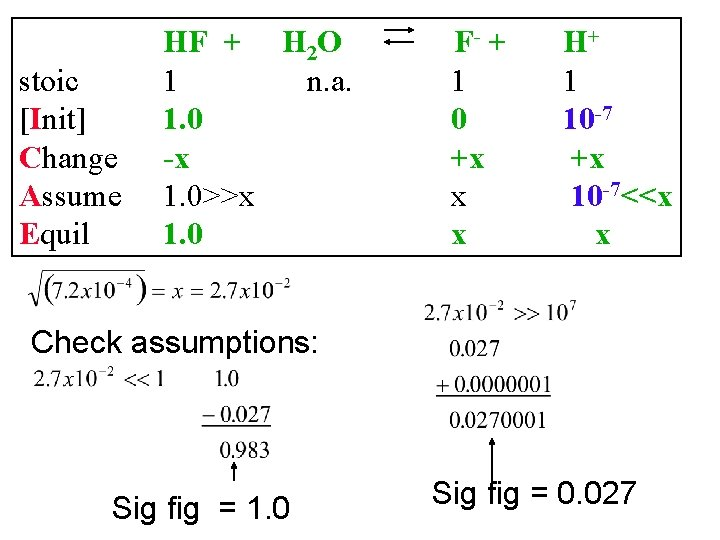stoic [Init] Change Assume Equil HF + H 2 O 1 n. a. 1. 0 -x 1. 0>>x 1. 0 F- + 1 0 +x x x H+ 1 10 -7 +x 10 -7<<x x Check assumptions: Sig fig = 1. 0 Sig fig = 0. 027stoic [Init] Change Assume Equil HF + H 2 O 1 n. a. 1. 0 -x 1. 0>>x 1. 0 F- + 1 0 +x x x H+ 1 10 -7 +x 10 -7<<x x Calculate the p. H of a solution that contains 1. 0 M HF and 1. 0 M HOC 6 H 5. Calculate the conc. of -OC 6 H 5 at this concentration.Example Calculations 1. HCl 2. Acetic acid (vinegar) 3. HF 4. B(OH)3 (Boric acid (eye wash)) (students Do this one yourself) 5. Mixture (HF and phenol) 6. Mixture (H 2 SO 4, HSO 4 -) 7. Triethylamine 8. Na. Acetate 9. Our heme exampleExample: calculate the p. H of 0. 0010 M sulfuric acid 1. Write down ALL possible reactions involving a proton 2. Excluding water, identify all the proton donors as 1. Strong acid a. Strong electrolyte: HNO 3, HCl, H 2 SO 4 (No Clean Socks) b. Give all strong acid protons to water or alpha dog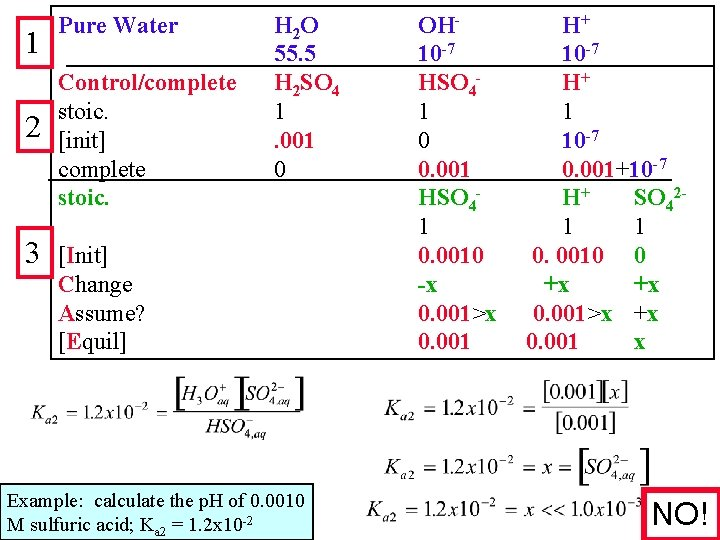1 2 3 Pure Water Control/complete stoic. [init] complete stoic. H 2 O 55. 5 H 2 SO 4 1. 001 0 [Init] Change Assume? [Equil] Example: calculate the p. H of 0. 0010 M sulfuric acid; Ka 2 = 1. 2 x 10 -2 OH 10 -7 HSO 41 0 0. 001 HSO 41 0. 0010 -x 0. 001>x 0. 001 H+ 10 -7 H+ 1 10 -7 0. 001+10 -7 H+ SO 421 1 0. 0010 0 +x +x 0. 001>x +x 0. 001 x NO!stoic. [Init] Change Assume? [Equil] HSO 41 0. 0010 -x 0. 001>x 0. 001 -x H+ 1 0. 0010 +x 0. 001>x 0. 001+x SO 421 0 +x +x x Here is our first example in which we can not Make assumptions Example: calculate the p. H of 0. 0010 M sulfuric acid; Ka 2 = 1. 2 x 10 -2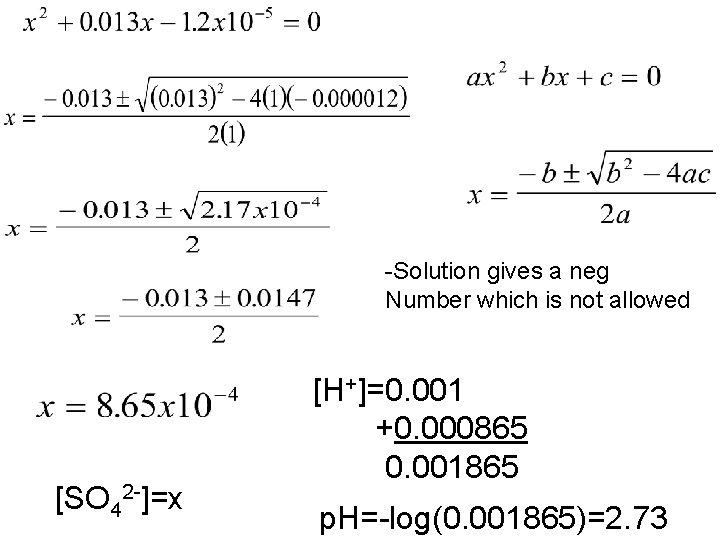-Solution gives a neg Number which is not allowed [SO 42 -]=x [H+]=0. 001 +0. 000865 0. 001865 p. H=-log(0. 001865)=2. 73Successive Approximations (iterations) Alternative Strategy to going to “exact equil. Expression” ITERATIVE SOLUTIONS Why? – because the real body or real world Is much too complex to always be able to Find an exact equilibrium expressionCalculate proton concentration of 0. 100 M HNO 2 using the iterative method (Ka=6. 0 x 10 -4) Pure Water H 2 O OHH+ 1 55. 5 10 -7 HNO 2 H+ stoic. 1 1 1 0 10 -7 2 [Init] Change -x +x +x Assum 0. 1>>x x x>>10 -7 [Equil] 0. 1 x x Calc 7. 7 x 10 -3 New Equil 0. 1 -7. 7 x 10 -3 x’’ 3 New calc 7. 44 x 10 -3 -3 x’’’ New Equil 0. 1 -7. 44 x 10 x’’’ 4 New new Calc 7. 45 x 10 -3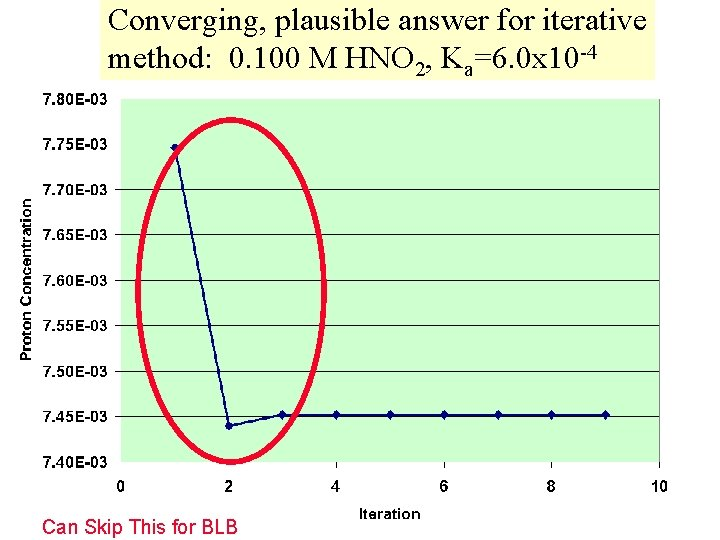Converging, plausible answer for iterative method: 0. 100 M HNO 2, Ka=6. 0 x 10 -4 Can Skip This for BLB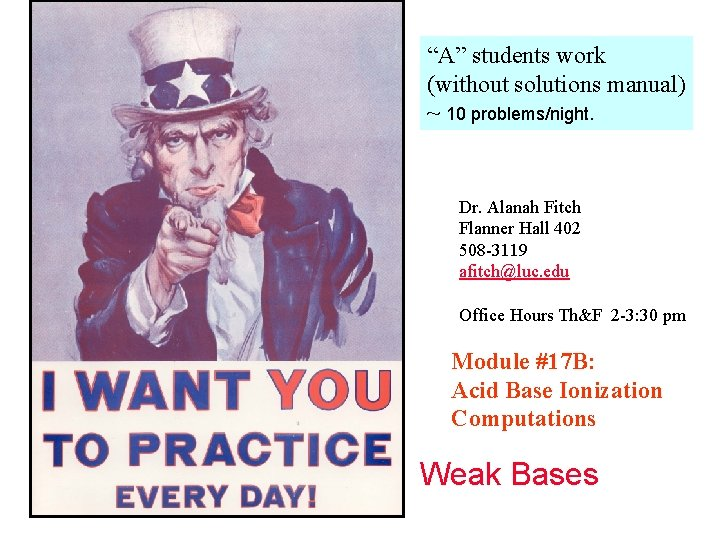“A” students work (without solutions manual) ~ 10 problems/night. Dr. Alanah Fitch Flanner Hall 402 508 -3119 [email protected] edu Office Hours Th&F 2 -3: 30 pm Module #17 B: Acid Base Ionization Computations Weak Bases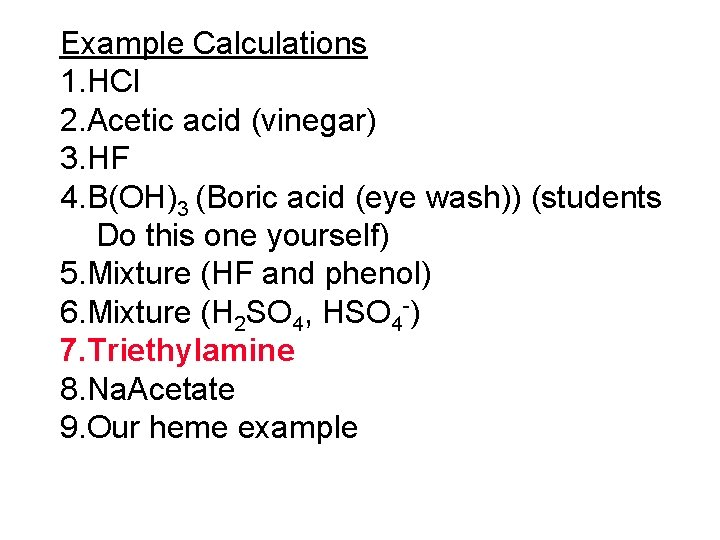Example Calculations 1. HCl 2. Acetic acid (vinegar) 3. HF 4. B(OH)3 (Boric acid (eye wash)) (students Do this one yourself) 5. Mixture (HF and phenol) 6. Mixture (H 2 SO 4, HSO 4 -) 7. Triethylamine 8. Na. Acetate 9. Our heme example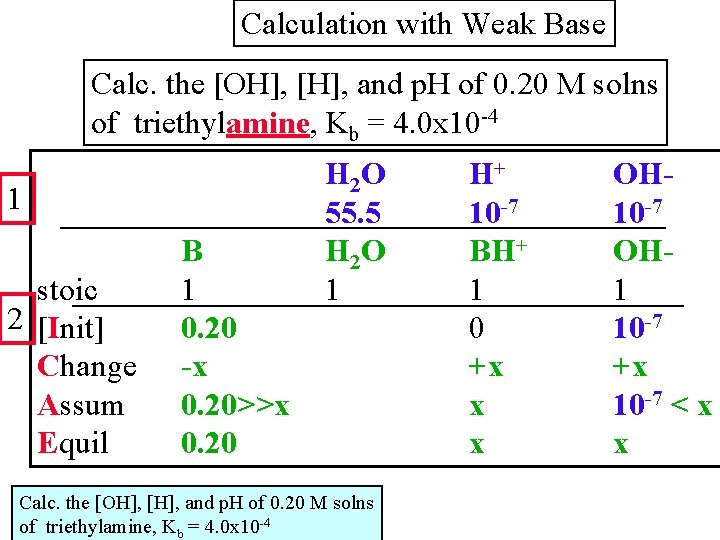Calculation with Weak Base Calc. the [OH], [H], and p. H of 0. 20 M solns of triethylamine, Kb = 4. 0 x 10 -4 1 stoic 2 [Init] Change Assum Equil B 1 0. 20 -x 0. 20>>x 0. 20 H 2 O 55. 5 H 2 O 1 Calc. the [OH], [H], and p. H of 0. 20 M solns of triethylamine, Kb = 4. 0 x 10 -4 H+ 10 -7 BH+ 1 0 +x x x OH 10 -7 OH 1 10 -7 +x 10 -7 < x x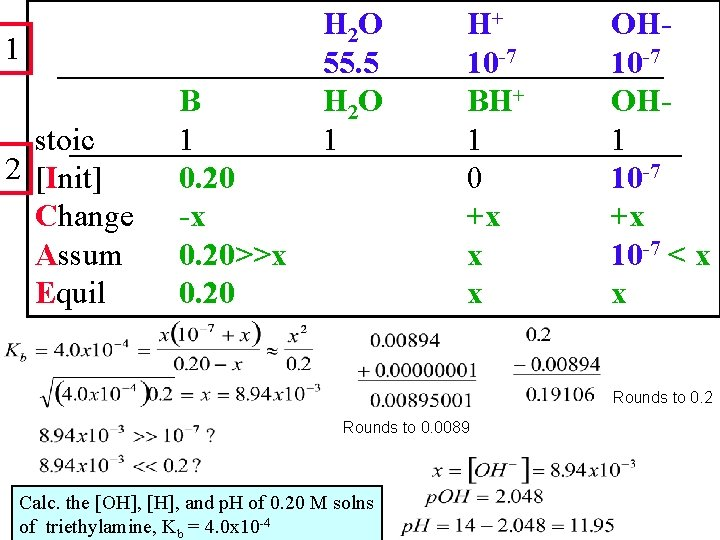1 stoic 2 [Init] Change Assum Equil B 1 0. 20 -x 0. 20>>x 0. 20 H 2 O 55. 5 H 2 O 1 H+ 10 -7 BH+ 1 0 +x x x OH 10 -7 OH 1 10 -7 +x 10 -7 < x x Rounds to 0. 2 Rounds to 0. 0089 Calc. the [OH], [H], and p. H of 0. 20 M solns of triethylamine, Kb = 4. 0 x 10 -4“A” students work (without solutions manual) ~ 10 problems/night. Dr. Alanah Fitch Flanner Hall 402 508 -3119 [email protected] edu Office Hours Th&F 2 -3: 30 pm Module #17 B: Acid Base Ionization Computations SaltsExample Calculations 1. HCl 2. Acetic acid (vinegar) 3. HF 4. B(OH)3 (Boric acid (eye wash)) (students Do this one yourself) 5. Mixture (HF and phenol) 6. Mixture (H 2 SO 4, HSO 4 -) 7. Triethylamine 8. Na. Acetate 9. Our heme exampleWrite all reactions involving protons, hydroxides Determine who is omega and will donate Hmm, a slight problem – we don’t know Kb If we place Na acetate in solution (to make a 0. 1 M solution) what are the main species present? What will be the p. H of the solution? Ka = 1. 8 x 10 -5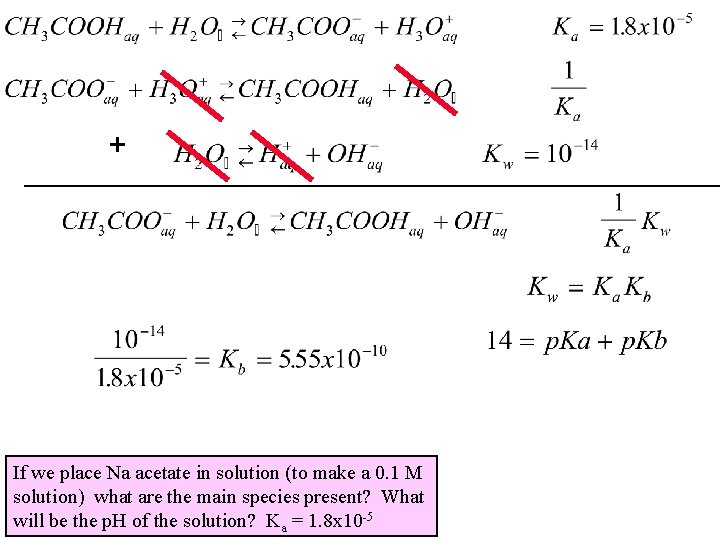+ If we place Na acetate in solution (to make a 0. 1 M solution) what are the main species present? What will be the p. H of the solution? Ka = 1. 8 x 10 -5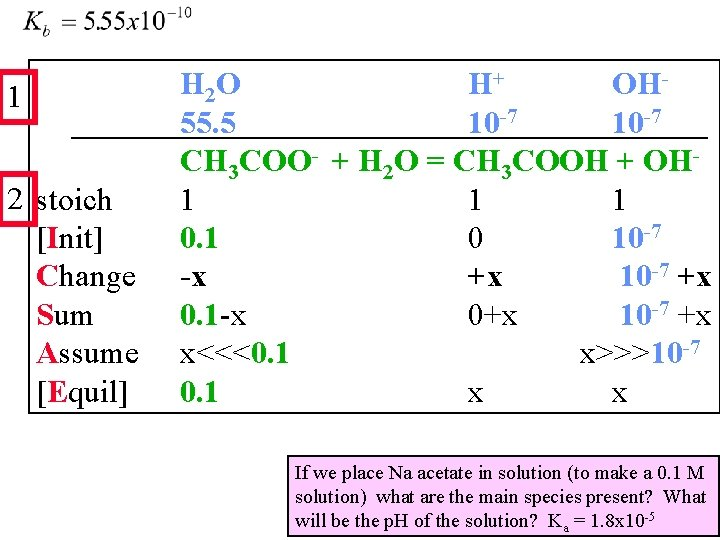1 2 stoich [Init] Change Sum Assume [Equil] H 2 O H+ OH 55. 5 10 -7 CH 3 COO- + H 2 O = CH 3 COOH + OH 1 1 1 0 10 -7 -x +x 10 -7 +x 0. 1 -x 0+x 10 -7 +x x<<<0. 1 x>>>10 -7 0. 1 x x If we place Na acetate in solution (to make a 0. 1 M solution) what are the main species present? What will be the p. H of the solution? Ka = 1. 8 x 10 -5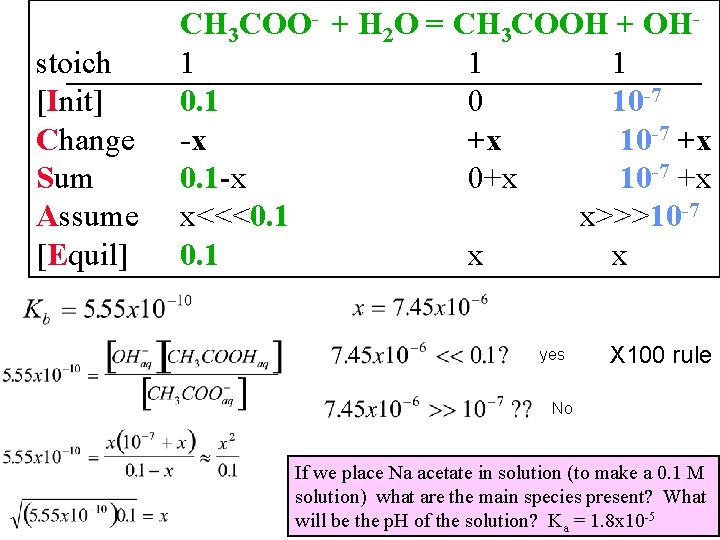stoich [Init] Change Sum Assume [Equil] CH 3 COO- + H 2 O = CH 3 COOH + OH 1 1 1 0 10 -7 -x +x 10 -7 +x 0. 1 -x 0+x 10 -7 +x x<<<0. 1 x>>>10 -7 0. 1 x x yes X 100 rule No If we place Na acetate in solution (to make a 0. 1 M solution) what are the main species present? What will be the p. H of the solution? Ka = 1. 8 x 10 -5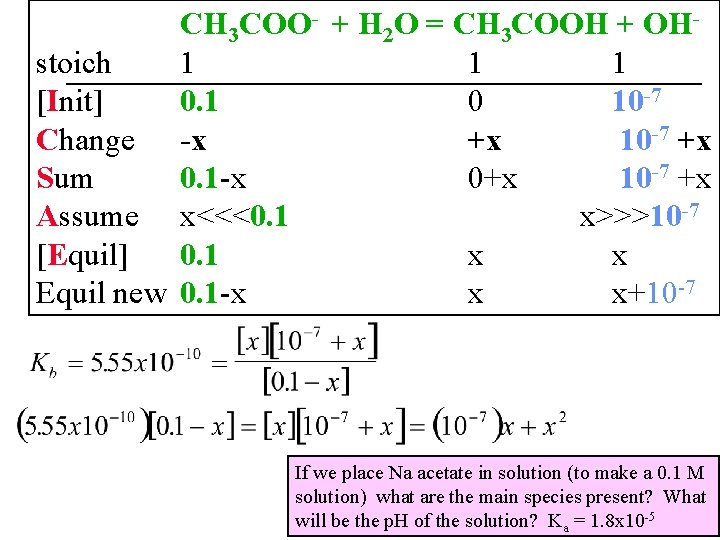CH 3 COO- + H 2 O = CH 3 COOH + OHstoich 1 1 1 [Init] 0. 1 0 10 -7 Change -x +x 10 -7 +x Sum 0. 1 -x 0+x 10 -7 +x Assume x<<<0. 1 x>>>10 -7 [Equil] 0. 1 x x Equil new 0. 1 -x x x+10 -7 If we place Na acetate in solution (to make a 0. 1 M solution) what are the main species present? What will be the p. H of the solution? Ka = 1. 8 x 10 -5Before we got 7. 45 x 10 -6“A” students work (without solutions manual) ~ 10 problems/night. Dr. Alanah Fitch Flanner Hall 402 508 -3119 [email protected] edu Office Hours Th&F 2 -3: 30 pm Module #17 B: Acid Base Ionization Computations Biological Chemistry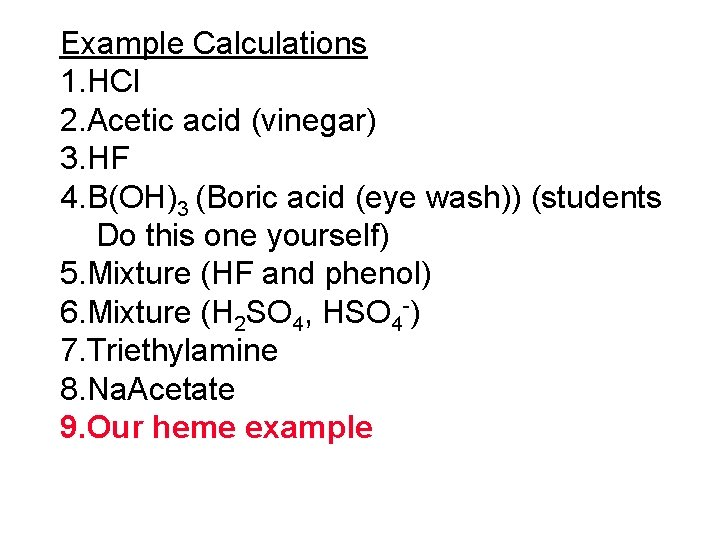Example Calculations 1. HCl 2. Acetic acid (vinegar) 3. HF 4. B(OH)3 (Boric acid (eye wash)) (students Do this one yourself) 5. Mixture (HF and phenol) 6. Mixture (H 2 SO 4, HSO 4 -) 7. Triethylamine 8. Na. Acetate 9. Our heme exampleProtein folding due to FRACTION of sites charged Hemeglobin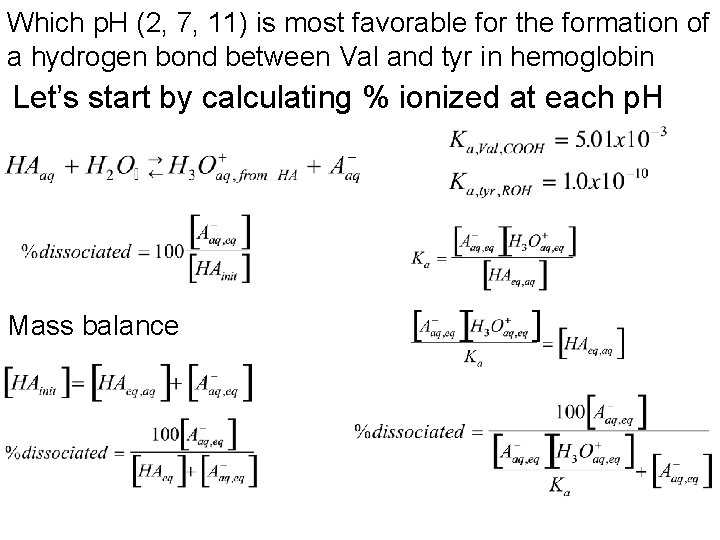Which p. H (2, 7, 11) is most favorable for the formation of a hydrogen bond between Val and tyr in hemoglobin Let’s start by calculating % ionized at each p. H Mass balanceWhich p. H (2, 7, 11) is most favorable for the formation of a hydrogen bond between Val and tyr in hemoglobin Repeat procedure With tyrosine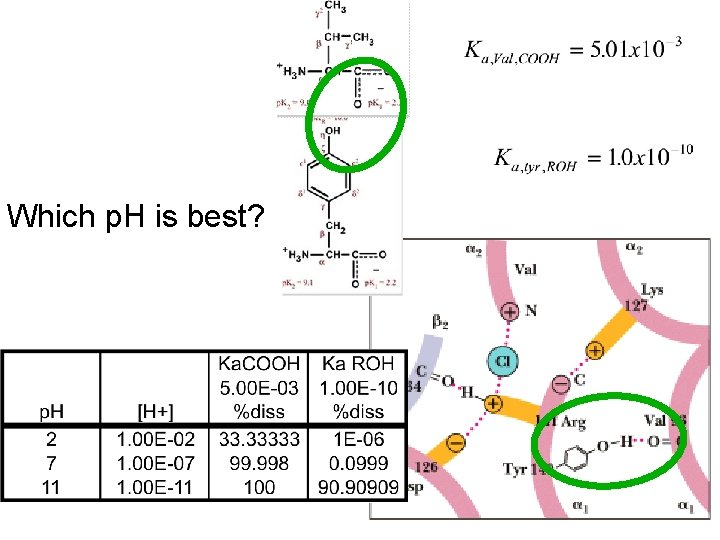Which p. H is best?“A” students work (without solutions manual) ~ 10 problems/night. Dr. Alanah Fitch Flanner Hall 402 508 -3119 [email protected] edu Office Hours Th&F 2 -3: 30 pm Module #17 B: Acid Base Ionization Computations